0%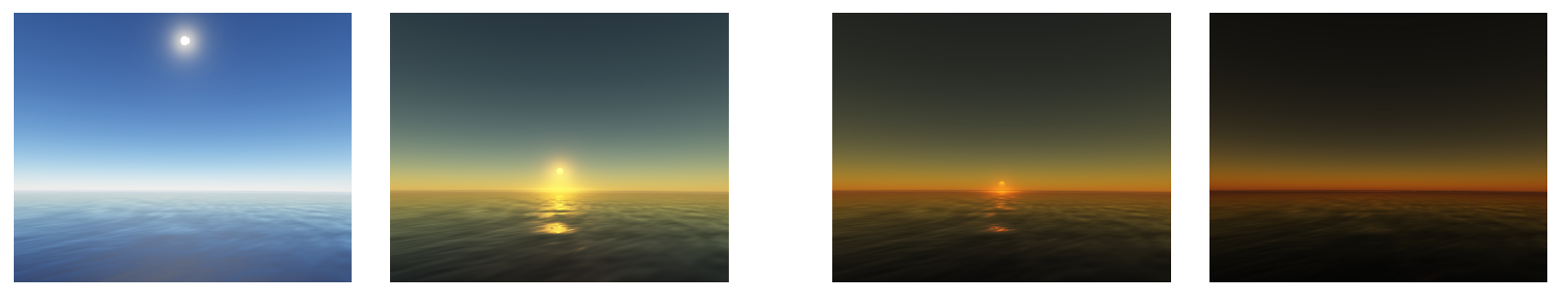##### 光散射

• 吸收（Absobption）： 参与介质把光能量转换为其他形式的能量。
• 外散射（Out-Scattering）： 改变光传播方向。
• 内散射（In-Scattering）：进入相机方向的入射辐射度。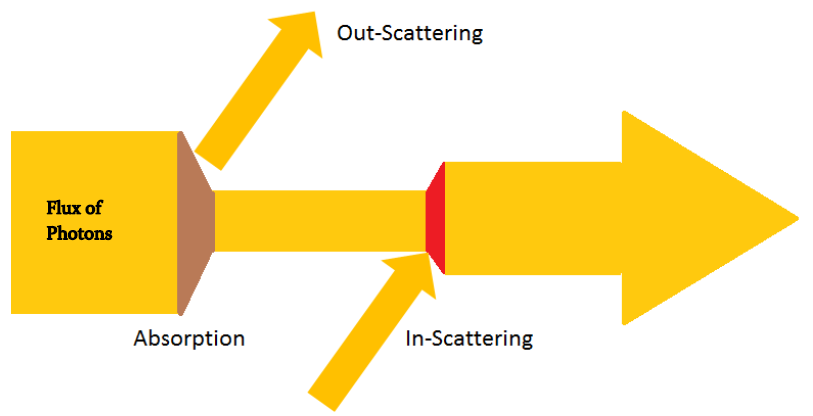##### 大气物理模型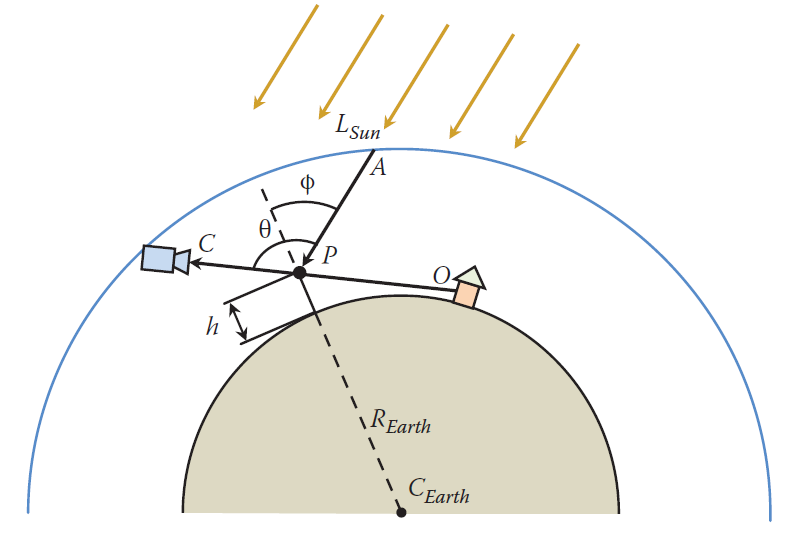* 角度：相机方向与太阳太阳的夹角。
* 高度：高度不同，粒子的密度值不同。
* 波长：波长较小的光分量更容易被散射。


##### 散射系数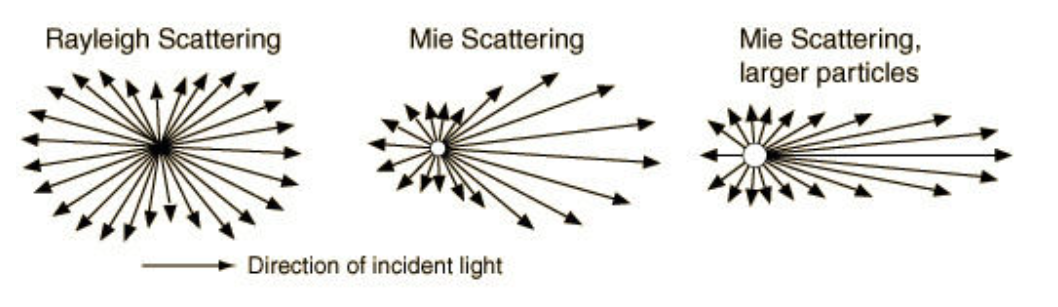###### 瑞利散射系数？

$\beta_{R}(\lambda) = 8\pi^3\frac{(n^2-1)^2}{3N_{e}\lambda^4}$

##### 相位函数

1. 瑞利相位函数
$F_{R}(\theta) = \frac{3}{4}(1+cos\theta)$

2. 米相位函数

$F_{M}(\theta, g) = \frac{3(1-g^2)}{2(2+g^2)}\frac{1+\cos^2(\theta)}{(1+g^2-2g\cos^2(\theta))^{3/2}}$

##### 密度函数

$p_{R,M}(h) = exp(-\frac{h}{H_{R,M}})$

##### 透射比函数

###### 光学深度$D(A->B) = \int_{A}^{B} (\beta_{R}^{e}e^{-h(t)/H_{R}} + \beta_{M}^{e}e^{-h(t)/H_{M}})dt$

• 瑞利散射消光系数: $\beta_{R}^{e} = \beta_{R}^{s}$，消光系数等于散射系数。
• 米散射消光系数： $\beta_{M}^{e} = \beta_{M}^{s} + \beta_{M}^{a}$，[BN08]论文中建议米散射吸收系数等于散射系数的0.1倍。

$T(A->B) = exp^{-D}$

##### 散射积分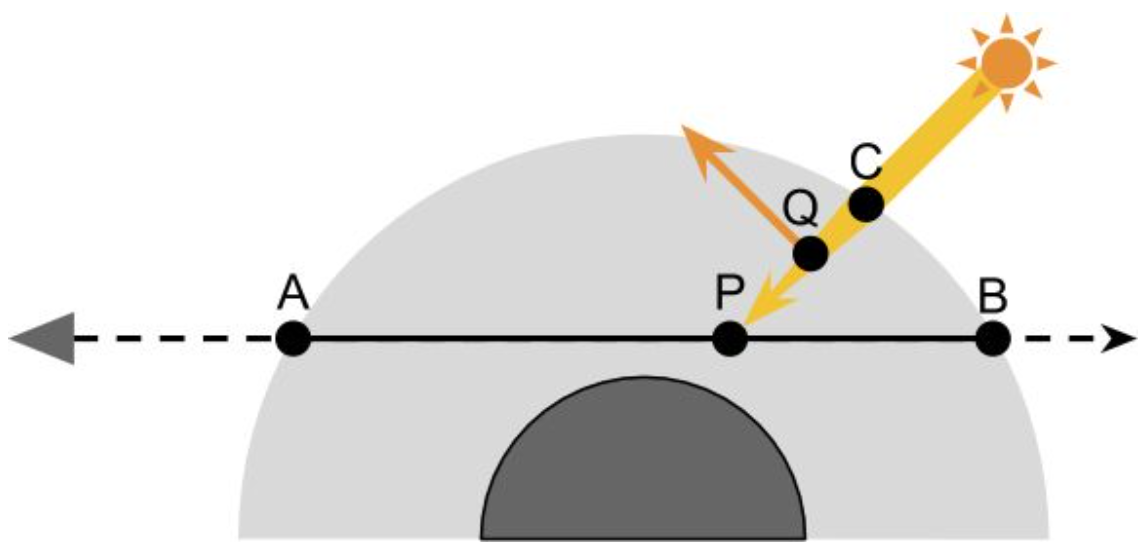$L_{In} = \int_{C}^{O}L_{Sun}e^{-T(A(s)->P(s))}e^{-T(P(s)->C)} * V(P(s))(\beta_{R}^{s}e^{-h(s)/H_{R}}p_{R}(\theta)+\beta_{M}^{s}e^{-h(s)/H_{M}}p_{M}(\theta)) ds$

###### 大气透视

$L = L_{O}e^{-T(O->C)} + L_{In}$

##### 参考资料
1. GPU Pro 5: High Performance Outdoor Light Scattering Using Epipolar Sampling.
2. Precomputed Atmospheric Scattering
3. Rendering Parametrizable Planetary Atmospheres with Multiple Scattering in Real-Time
4. Blue Sky
5. 基于物理的大气渲染
6. 基于体积的大气散射